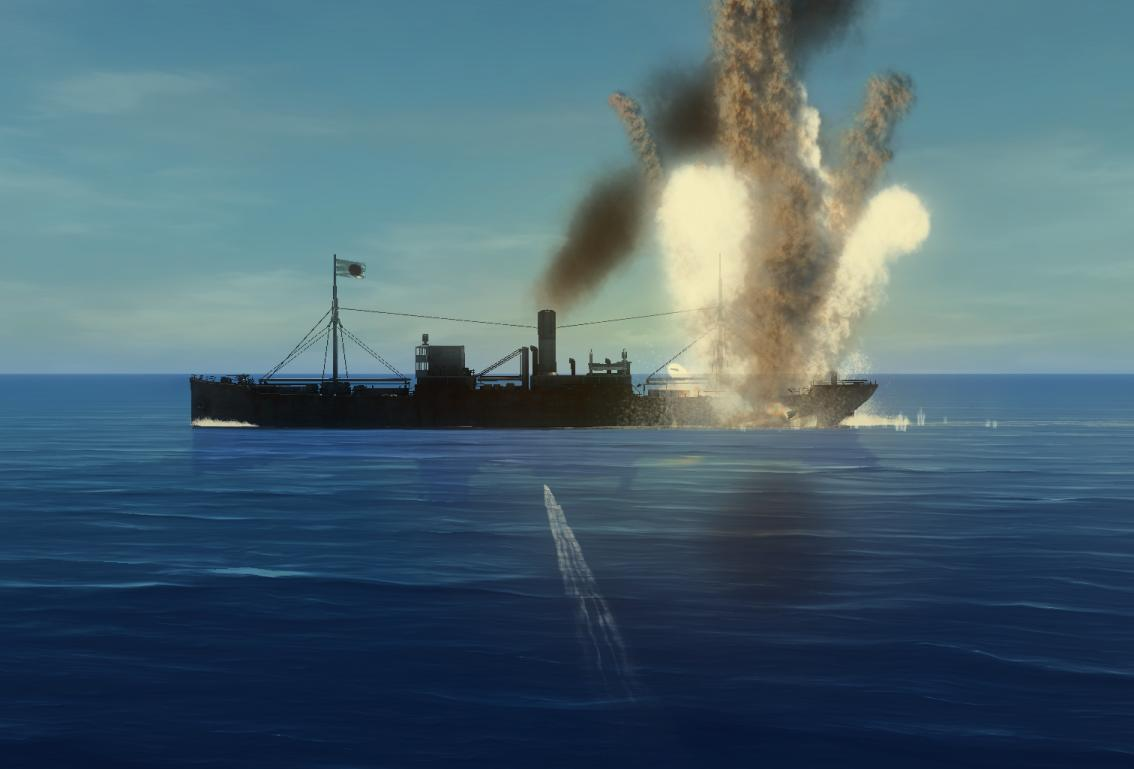# Torpedoes Sinking A Ship

Probability Level 2Suppose that each torpedo has a 1/3 probability of sinking a ship, 1/3 probability of damaging it and 1/3 of missing it altogether. Moreover, 2 damaging shots will also sink the ship. The probability that firing four torpedoes will sink the ship can be expressed as $\frac{a}{b}$, where $a$ and $b$ are coprime, positive integers. Calculate $a+b$.

Note: All that we care about is that the ship is sunk at the end of the 4 shots. The first shot could already have sunk the ship.

×